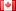# Revision history [back]

First off, what is missing here (but is in your worksheet) is var(r) at the beginning. Otherwise your first one is calling R!

As to the others, as you see on the worksheet, the difference is that the first one is a "symbolic expression", in which variables have to be substituted, while the second is a "callable symbolic expression", where you don't have to do this. Compare:

sage: f1.subs(r=3)
f(3)
sage: f2(3)
f(3)

sage: preparse("f2(r) = function('f', r)")
'__tmp__=var("r"); f2 = symbolic_expression(function(\'f\', r)).function(r)'


What happens is that the .function method turns it into something with a specific variable r that is the input. If you had 'constants' like 'a' or 'b' in your function, this would make more sense, e.g.

sage: var('a,b,c')
(a, b, c)
sage: f3(r)=a*b*c*function('f',r)
sage: f3
r |--> a*b*c*f(r)


So you could only substitute r with the function syntax; for the rest you'd need the .subs method.

Otherwise, they should be pretty much identical, I think. You have to be a little careful with whether you call the second one f2(r) or just f2, I think, in things like numerical integration, maybe. I can't remember the exact contexts in which that matters, but it is not frequent.2 No.2 RevisionDSM5242 ●21 ●68 ●112

First off, what is missing here (but is in your worksheet) is var(r)var("r") at the beginning. Otherwise your first one is calling R!

As to the others, as you see on the worksheet, the difference is that the first one is a "symbolic expression", in which variables have to be substituted, while the second is a "callable symbolic expression", where you don't have to do this. Compare:

sage: f1.subs(r=3)
f(3)
sage: f2(3)
f(3)

sage: preparse("f2(r) = function('f', r)")
'__tmp__=var("r"); f2 = symbolic_expression(function(\'f\', r)).function(r)'


What happens is that the .function method turns it into something with a specific variable r that is the input. If you had 'constants' like 'a' or 'b' in your function, this would make more sense, e.g.

sage: var('a,b,c')
(a, b, c)
sage: f3(r)=a*b*c*function('f',r)
sage: f3
r |--> a*b*c*f(r)


So you could only substitute r with the function syntax; for the rest you'd need the .subs method.

Otherwise, they should be pretty much identical, I think. You have to be a little careful with whether you call the second one f2(r) or just f2, I think, in things like numerical integration, maybe. I can't remember the exact contexts in which that matters, but it is not frequent.